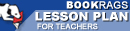Lesson Plans
Related Topics

# Daily Lessons for Teaching The Light Fantastic

This set of Lesson Plans consists of approximately 125 pages of tests, essay questions, lessons, and other teaching materials.
 View a FREE sample## Objective

Objective: The introduction in a book is meant to grab the reader's attention. The objective of this lesson is to draw attention to the introduction in a story.

1) Discussion: Ask the students to discuss the introduction in The Light Fantastic. What does the author do in order to get the reader's attention? Explain.

2) Classwork: Give the students a list of first lines or introductions from literature that are well known. See how many of the students can identify where these different examples came from. Have the students comment on why the lines are gripping and what the author used to make these introductions attention grabbing.

3) Homework: Have the students write a short introduction to a unique work of their own. It can be for fiction or non-fiction or it can be an introduction with no story line in mind. Have the students comment on which ones are...

 This section contains 4,765 words (approx. 16 pages at 300 words per page) View a FREE sample